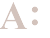## Calculate Your Daily Calorie Needs

### Question:

How can I calculate how many calories I need daily?Try the following equation, which is designed specifically for women and accepted by nutritionists around the world. It takes into account factors such as your height, weight, age and exercise habits, and gives a fairly accurate evaluation.

To find out how many calories your body needs daily, just insert your current weight and height into the equation. If you're trying to shed pounds, use your goal weight instead to figure out your desired calorie count.

Steps:

1. Multiply your current weight in pounds by 4.5.���_____
2. Multiply your height in inches by 15.88.���_____
3. Add the numbers in steps (1) and (2).���_____
4. Multiply your age by 5.���_____
5. Subtract step (4) from step (3).���_____
6. Subtract 161 from step (5).���_____
7. Multiply step (6) by your activity factor*.���_____

1.2 -- little activity or none
1.375 -- light activity one to three days a week
1.55 -- moderate three to five days a week
1.725 -- intense at least six days a week
1.9 -- intense exercise plus physical job

Like This?